WEBINAR
Understanding Eccentricity Effects on Stator Tooth Forces for eNVH Analysis
Thursday, June 8, 2023
Time
SESSION 1
SESSION 2
CEST (GMT +2)
03:00 PM
08:00 PM
EDT (GMT -4)
09:00 AM
02:00 PM
HOME / Applications / Double-Sided Flat PM Linear Synchronous Motor Analysis Using EMWorks2D

# Double-Sided Flat PM Linear Synchronous Motor Analysis Using EMWorks2D

Used Tools:## Overview

The increase in the demand of linear motors is principally driven by the replacement of traditional mechanical (ball screws, gear trains, cams), hydraulic, or pneumatic linear motion systems in manufacturing processes with direct electromechanical drives. Linear motors are also used in various applications such as high-speed maglev transport and elevator hoisting.

Linear synchronous motors (LSMs) can be classified as follows,
• Flat (planar) or Tubular (cylindrical)
• Single-sided or Double-sided
• Slotted or Slotless
• Iron-cored or Air-cored
• Transverse-flux or Longitudinal-flux

In this application note a 3-phase double-sided FLSM motor prototype, illustrated in Figure 1, will be analyzed using EMWorks2D inside Solidworks.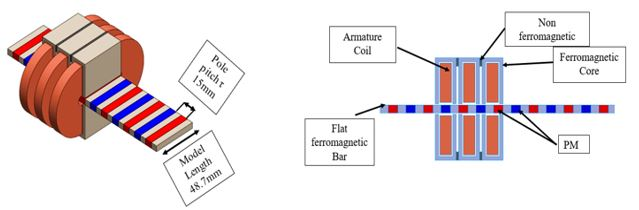Fig. 1. Flat Slotted Iron Cored Linear Synchronous Motor (FLSM) Model

## FLSM Settings

### 1. Material Selection

The materials used in the FLSM model are shown in Table 1. The stainless steel 1010 is used for the core ferromagnetic material and the rare-earth sintered NdFeB grade-35 characterized with high coercivity and remanence are used as magnets. Hence, this would highly improve the performance of the motor.

Motor Componen Material
Armature coil Copper
Flat ferromagnetic bar Stainless steel 1010
Ferromagnetic core Stainless steel 1010
Permanent magnet (PM) N35
Non ferromagnetic core Aluminum
Table 1. FLSM Material

### 2. Coercivity Direction and Winding Layout

As shown in Figure 2, the coercivity direction is chosen so that the magnetic flux will be longitudinal from the magnets, the ferromagnetic flat bars will play the role of a magnetic flux conducting material so they will transform the direction of flux to be transversal if a ferromagnetic core is sufficiently close to them.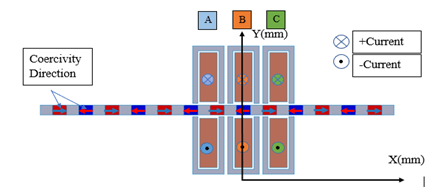Fig. 2. Coercivity Direction and Winding Layout

### 3. Defining Distance for Linear Motion

The distance is defined as a mate between the mover (magnets + ferromagnetic bars) and the stationary component, which is in this case the armature (ferromagnetic core + armature winding) as shown in Figure 3.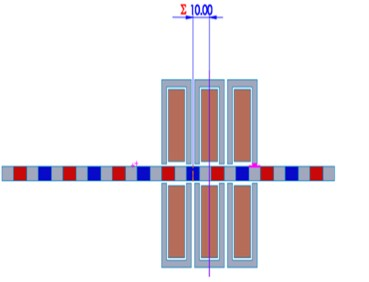Fig. 3. Distance Definition

## Simulation and results

### 1. Open circuit simulation of FLSM (Detent force and Back EMF)

To perform an open circuit or no-load simulation, the armature winding should be set to current-driven mode inside the EMWorks2D winding editor and set the current magnitude to zero, the simulation time and time step are chosen so that the mover displacement is equal to two times the  pole pitch. The other parameters are listed as shown in Table 2.

Parameter Value
Simulation time 0.03 s
Time step 0.0005 s
Velocity 1 m/s
Distance 30 mm
Initial position 0 mm
Number of turns per phase 280
Table 2. Open Circuit Simulation Settings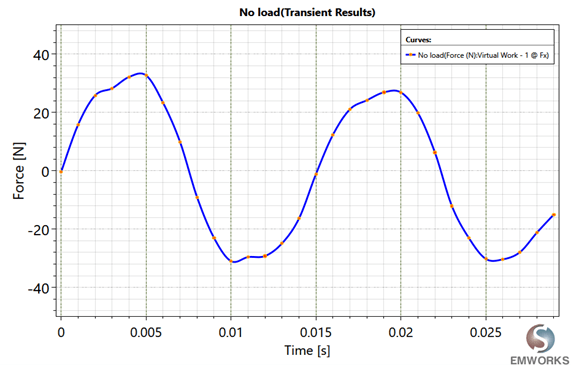Fig. 4. Detent Force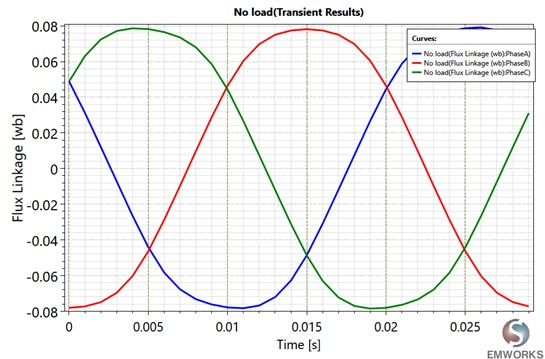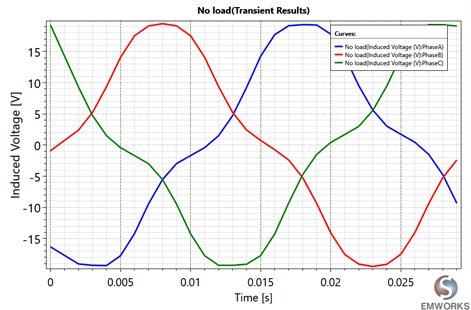Fig. 6. Induced Voltage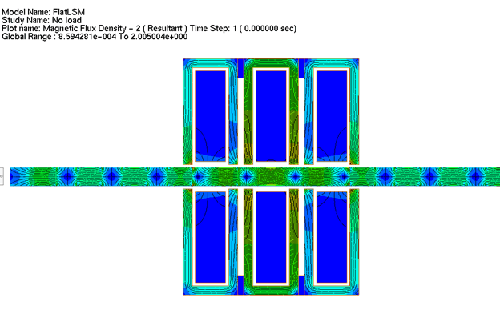Fig. 7. Flux Density

### 2. On-load analysis of FLSM (Thrust Values)

For the on-load test, the applied current values depend on the distance value(x) of the mover, the moving range will be set to a pole pitch value of 15 mm and the peak current value is 8 A, linear velocity will be set to 1 m/s. The current equations for all three phases are as shown below,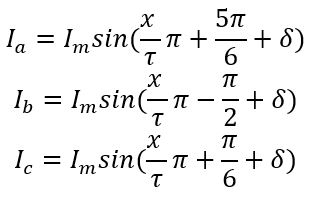Where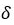is the load angle, to get the maximum thrust, different simulations were performed with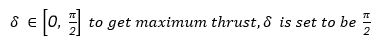Linear velocity depends only on frequency and pole pitch value according to the equation,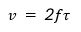Similarly, the values of the current are obtained at a 33Hz frequency.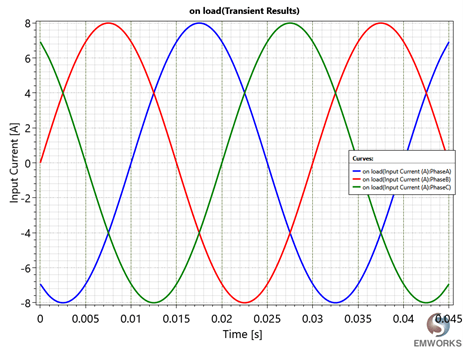Fig. 8. Input Current Versus Distance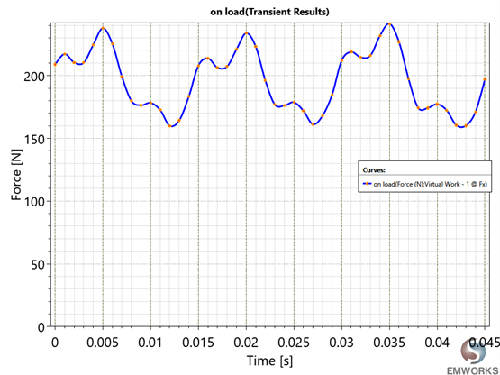Fig. 9. Thrust

The simulation results obtained are tabulated as shown in Table 3.

Parameter Value
Maximum force, fmax 241.34 N
Minimum force, fmin 160.01 N
Average force, fav 196.10 N
Force ripple coefficient, kr 0.414
Maximum detent cogging force 25.66 N
Table 3. Simulation Results

The force ripple coefficient is computed as: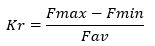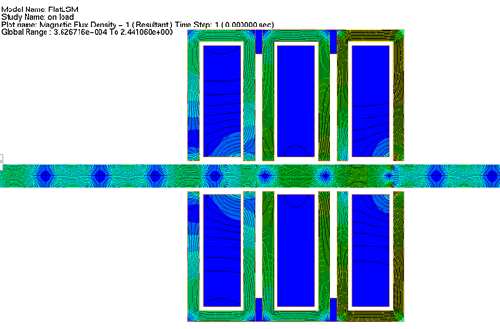Fig. 10. On- Load Flux Density Plot

### 3. Dynamic Simulation of FLSM

The FLSM dynamic simulation parameters are shown in Table 4. The armature currents are the same as the on-load simulation and the speed values are computed using the dynamic Newtonian equation

Parameter Value
Mover mass (kg) 1
Dumping Factor (N-s/m) 0.001
Initial velocity (m/s) 0
Initial position (m) 0
Table 4. FLSM Dynamic Simulation Parameters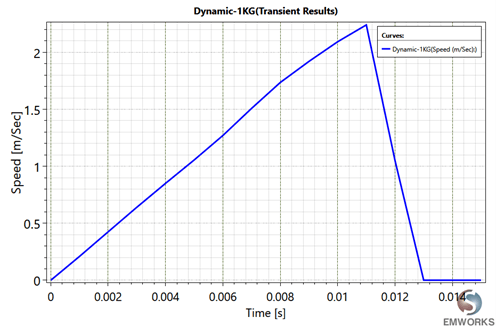Fig. 11. Dynamic Speed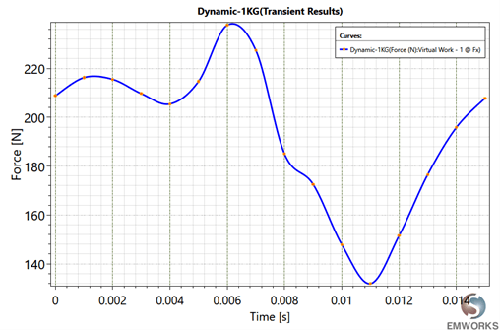Fig. 12. Dynamic Force

The simulation results obtained are tabulated as shown in Table 5.

Parameter Value
Maximum force, fmax 237 N
Minimum force, fmin 132 N
Average force, fav 194.04 N
Force ripple coefficient, kr 0.5411
Table 5. Simulation Results

#### Reference

 Gieras, Jacek F., Zbigniew J. Piech, and Bronislaw Tomczuk. Linear synchronous motors: transportation and automation systems. CRC press, 2016.